# Find a cone in R2 that is not convex, Prove that a subset X of Rr is a convex cone if and only if x,y eX implies that X... related homework questions

• #### Find a cone in R2 that is not convex, Prove that a subset X of Rr is a convex cone if and only if x,y eX implies that X...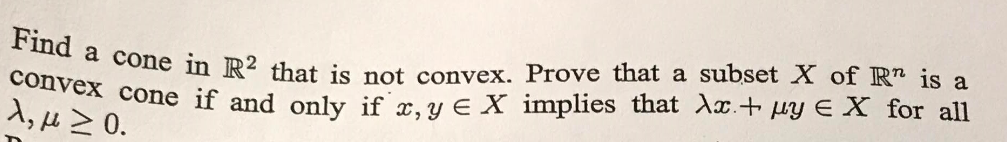Find a cone in R2 that is not convex, Prove that a subset X of Rr is a convex cone if and only if x,y eX implies that Xx+ py E X for all Find a cone in R2 that is not convex, Prove that a subset X of Rr is a convex cone if and only if x,y eX...

• #### I have to make a true statement with this question: {All states west of the Rocky Mountains} {All states west of the Atlantic Ocean} I have to determine if the two statements are: Proper Subset is a subset of is not a subset of proper subset and is

I have to make a true statement with this question:{All states west of the Rocky Mountains} {All states west of theAtlantic Ocean}I have to determine if the two statements are: Proper Subsetis a subset ofis not a subset ofproper subset and is a subset of

• #### p implies r q implies r conclusion (p or q ) implies r show they sre...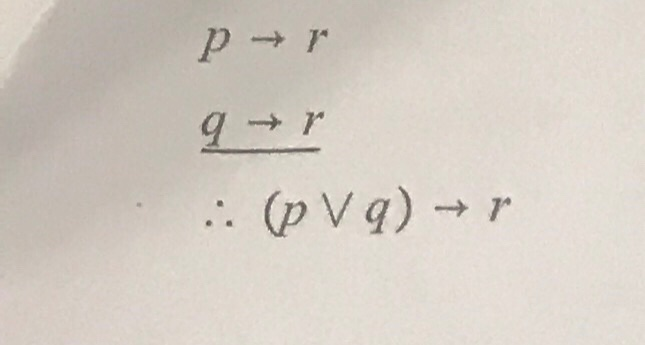p implies r q implies r conclusion (p or q ) implies r show they sre logical equivalent (pVa) (pVa)

• #### If A is a subset of B (not necessarily proper) and B is a subset of C (not necessarily proper) the A is a proper subset of C

If A is a subset of B (not necessarily proper) and B is a subset of C (not necessarily proper) the A is a proper subset of C.True or Falseif false must provide a counterexample

• #### If A is a subset of B (not necessarily proper) and B is a subset of C (not necessarily proper) the A is a proper subset of C

If A is a subset of B (not necessarily proper) and B is a subset of C (not necessarily proper) the A is a proper subset of C. True or Falseif false must provide a counterexample

• #### CANCELLATION LAWS: Let G be a group and let a, b, c E G. Then . ab = ac implies b = c, and ba ca implies b-c 1. Prove the two Cancellation Laws 2. Choose an integer n > 12 for which Zn is not...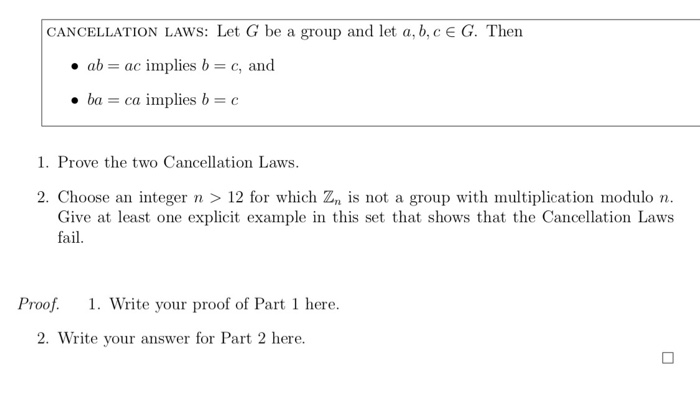CANCELLATION LAWS: Let G be a group and let a, b, c E G. Then . ab = ac implies b = c, and ba ca implies b-c 1. Prove the two Cancellation Laws 2. Choose an integer n > 12 for which Zn is not a group with multiplication modulo n Give at least one explicit example in this...

• #### Need help with this subset problem Consider S aa, ba and T- {a, b}. Show that S is a proper subset of T. Consider S aa, ba and T- {a, b}. Show that S is a proper subset of T.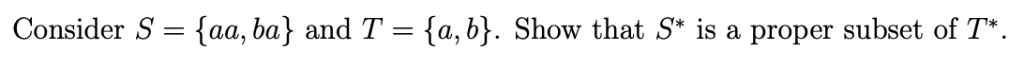Need help with this subset problem Consider S aa, ba and T- {a, b}. Show that S is a proper subset of T. Consider S aa, ba and T- {a, b}. Show that S is a proper subset of T.

• #### If A is a subset of B and B is a subset of C, can you conclude that A is a subset of C

If A is a subset of B and B is a subset of C, can you conclude that A is a subset of C? Can you conclude that A is a proper subset of C? If A is a proper subset of B and B is a proper subset of C, can you conclude that A is a subset of...

• #### blem 4 rove that surjectivity implies the range and udomain are equal for a wiven Problem 5 Prove the following blem 4 rove that surjectivity implies the range and udomain are equal for a wi...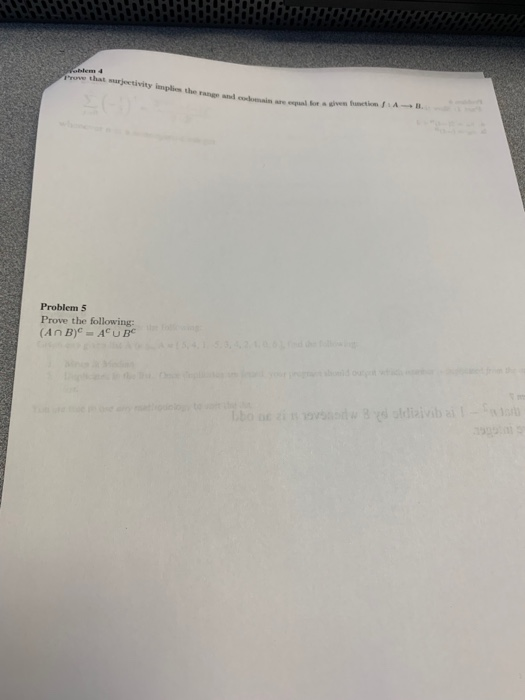blem 4 rove that surjectivity implies the range and udomain are equal for a wiven Problem 5 Prove the following blem 4 rove that surjectivity implies the range and udomain are equal for a wiven Problem 5 Prove the following

• #### MATLAB ONLY MATLAB ONLY MATLAB ONLY MATLAB ONLY MATLAB ONLY MATLAB ONLY MATLAB ONLY MATLAB ONLY....... Project V: Fourier Based System Modeling The last project in the class involves the modeling of a...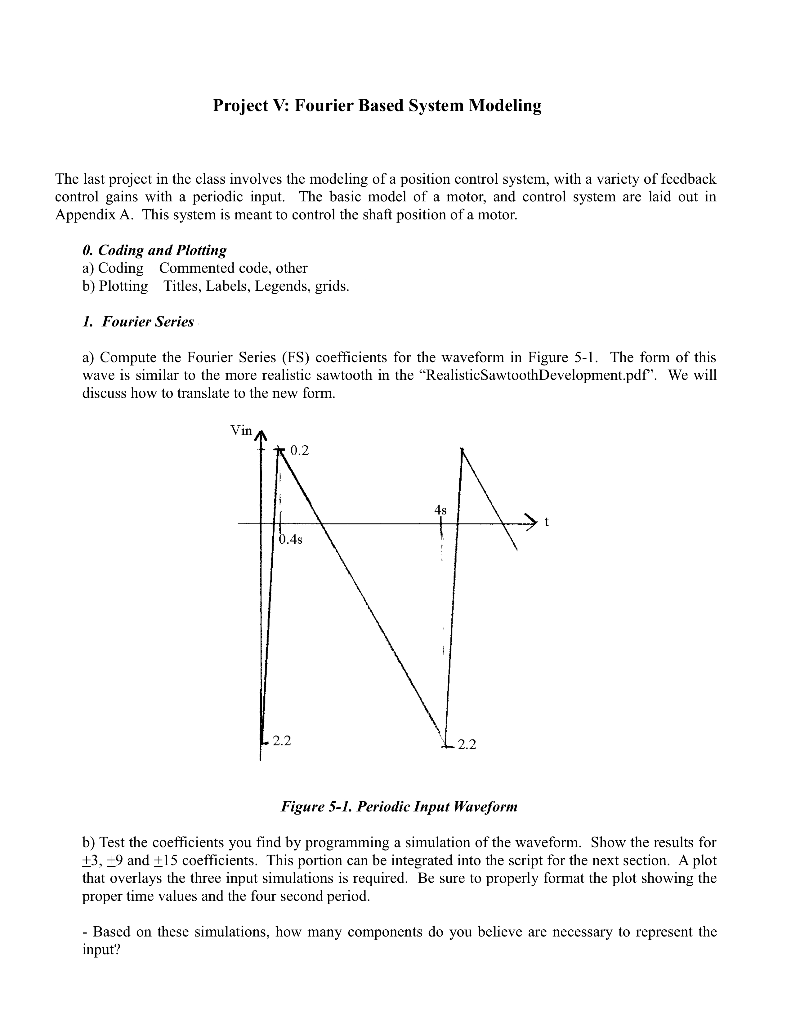MATLAB ONLY MATLAB ONLY MATLAB ONLY MATLAB ONLY MATLAB ONLY MATLAB ONLY MATLAB ONLY MATLAB ONLY....... Project V: Fourier Based System Modeling The last project in the class involves the modeling of a position control system, with a variety of fcedback control gains with a periodic input. The basic model of a motor, and control system are laid out in...

• #### If inflation falls unexpectedly, this implies QUESTION 9 If inflation falls unexpectedly, this implies a redistribution...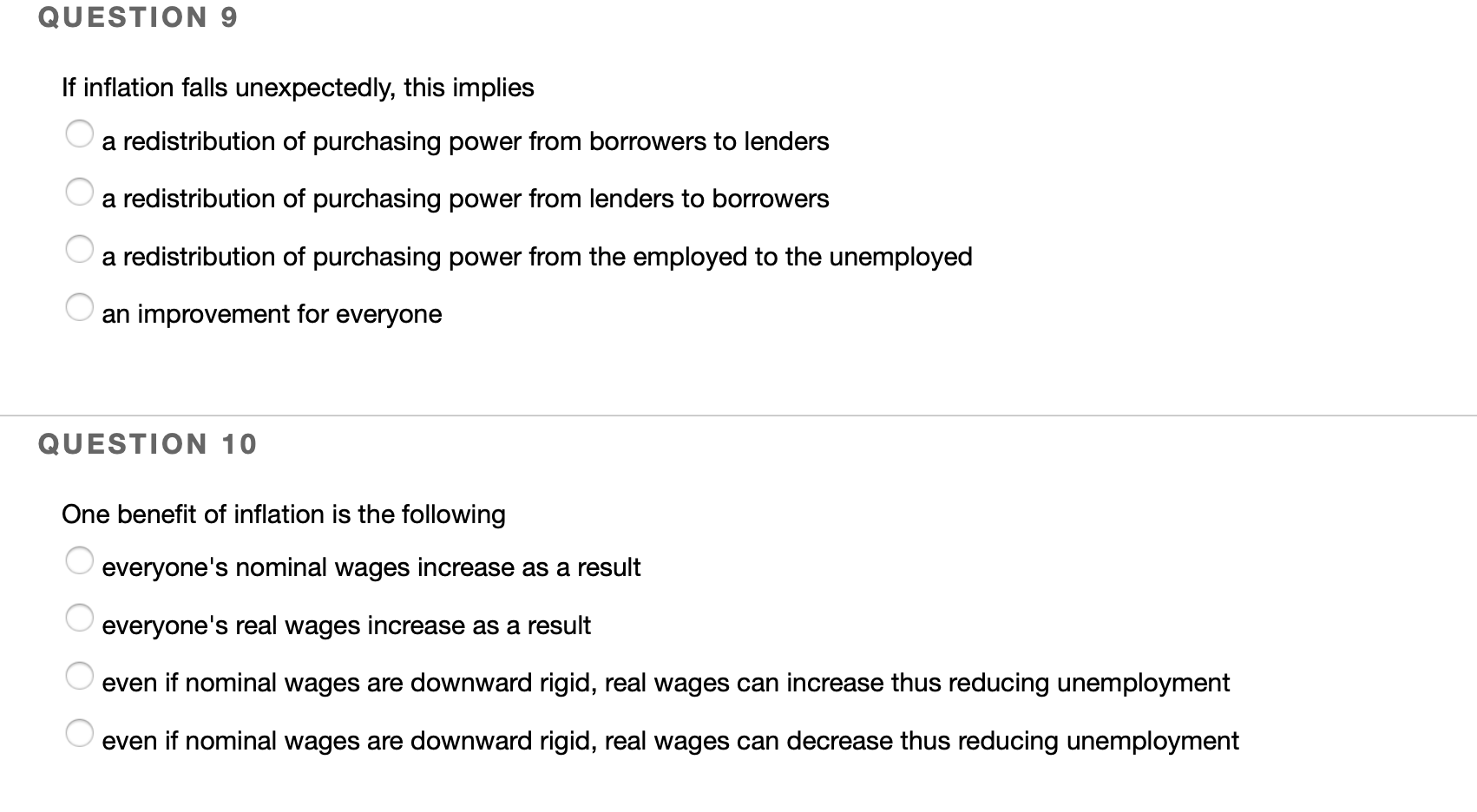If inflation falls unexpectedly, this implies QUESTION 9 If inflation falls unexpectedly, this implies a redistribution of purchasing power from borrowers to lenders a redistribution of purchasing power from lenders to borrowers a redistribution of purchasing power from the employed to the unemployed O an improvement for everyone QUESTION 10 One benefit of inflation is the following everyone's nominal wages...

• #### What David Ricardo saw was thát it could still be mutually beneficial for both countries to...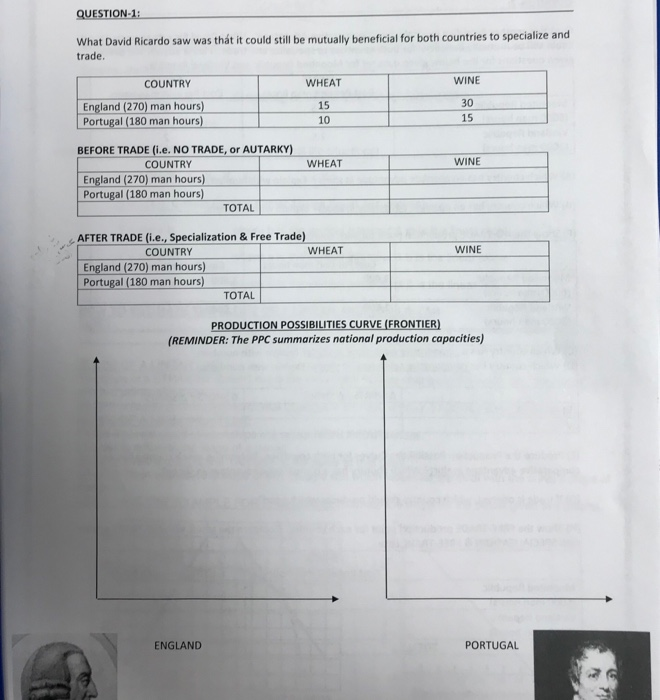What David Ricardo saw was thát it could still be mutually beneficial for both countries to specialize and trade COUNTRY WHEAT WINE England (270) man hours) Portugal (180 man hours 15 10 30 15 BEFORE TRADE (i.e. NO TRADE, or AUTARKY) COUNTRY WHEAT WINE England (270) man hours) Portugal (180 man hours) TOTAL COUNTRY WHEAT WINE England (270) man hours)...

• #### R is convex over C. Show that 4) Assume that is a convex subset of IR"...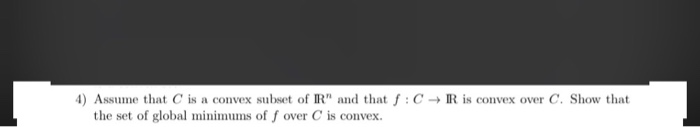R is convex over C. Show that 4) Assume that is a convex subset of IR" and that f: the set of global minimums of over C is convex.

• #### Question 3 (Chapter 6) 13+2+3+6 14 marks Fix p EN and consider the following set: : T1 (a) Prove that Cp is convex. (b) Prove that C, is a cone. (c) Compute Ci and C2. (d) Show that x = 0 is an e...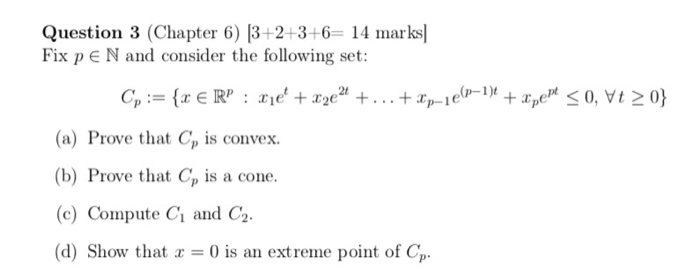Question 3 (Chapter 6) 13+2+3+6 14 marks Fix p EN and consider the following set: : T1 (a) Prove that Cp is convex. (b) Prove that C, is a cone. (c) Compute Ci and C2. (d) Show that x = 0 is an extreme point of CP. Question 3 (Chapter 6) 13+2+3+6 14 marks Fix p EN and consider the...

• #### In multiple regression, rejecting the null hypothesis in the F-test implies that... (a) a subset of...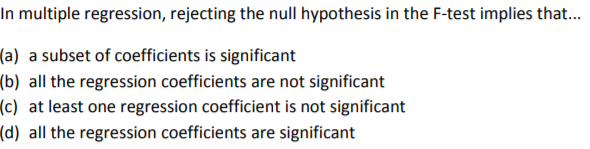In multiple regression, rejecting the null hypothesis in the F-test implies that... (a) a subset of coefficients is significant (b) all the regression coefficients are not significant (c) at least one regression coefficient is not significant (d) all the regression coefficients are significant

• #### Running average of a convex function. Suppose fR R is convex, with R+ S dom f. Show that its running average F, defined as F(a)-f(t) dt. dom F-R++ 2 0 is convex. You can assume f is differentiable....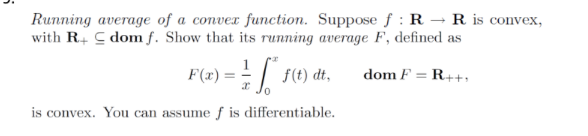Running average of a convex function. Suppose fR R is convex, with R+ S dom f. Show that its running average F, defined as F(a)-f(t) dt. dom F-R++ 2 0 is convex. You can assume f is differentiable. Running average of a convex function. Suppose fR R is convex, with R+ S dom f. Show that its running average F,...

• #### ) For sets A and B, determine whether A = B, A is a subset of B, or B is a subset of A A = {x| x є N and 13 < x < 20} B = {14, 15, 16, 17, 18}

) For sets A and B, determine whether A = B, A is a subset of B, or B is a subset of A A = {x| x є N and 13 < x < 20} B = {14, 15, 16, 17, 18}

• #### suppose c is a subset of d and d is a subset of c

suppose c is a subset of d and d is a subset of c. if n(c) = 5, find n (d).

Free Homework App